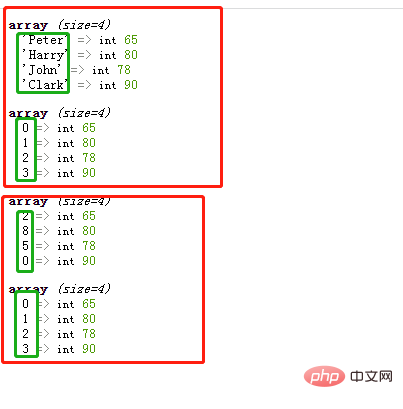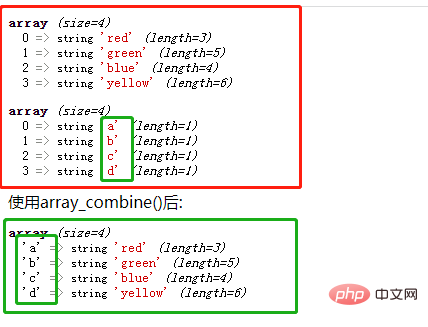# php怎么改变数组key的值php改变数组key的值，即改变数组的键名。有两种方法：

• 使用array_values()函数

• 使用array_combine()函数

array_values() 函数可以获取数组中所有元素的值，该函数语法格式如下：

`array_values(\$array)`
• 参数 \$array 为被操作的数组。

array_values() 函数特别适合用于数组中元素下标混乱的数组，或者关联数组。

array_values() 函数可以返回一个包含给定数组中所有值的数组，但不保留键名。即被返回的数组将使用索引数组的形式，数组的索引从 0 开始且以 1 递增。

```<?php
\$arr1=array("Peter"=>65,"Harry"=>80,"John"=>78,"Clark"=>90);
var_dump(\$arr1);
var_dump(array_values(\$arr1));

\$arr2=array(2=>65,8=>80,5=>78,0=>90);
var_dump(\$arr2);
var_dump(array_values(\$arr2));
?>```array_combine() 函数通过合并两个数组来创建一个新数组，其中的一个数组是键名，另一个数组的值为键值。

`array_combine(\$keys,\$values);`
• \$keys 必需。键名数组。

• \$values 必需。键值数组。

```<?php
\$arr=array("red","green","blue","yellow");
var_dump(\$arr);
\$keys=array("a","b","c","d");
var_dump(\$keys);
echo "使用array_combine()后:";
\$arr=array_combine(\$keys,\$arr);
var_dump(\$arr);
?>```• 相关标签：php数组 php
• 相关文章

相关视频

作者信息